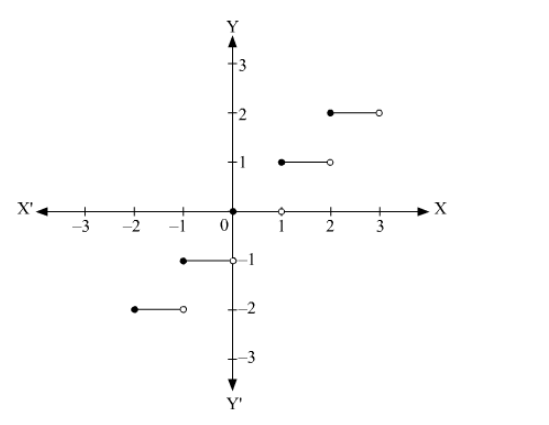# The function f(x) = [x] is continuous at

Question:

The function f(x) = [x] is continuous at

(a) 4

(b) $-2$

(c) 1

(d) $1.5$

Solution:

The graph of f(x) = [x] is shown below.It can be seen that, the function f(x) = [x] is discontinuous at all integral values of x. It is continuous at all points except the integer points.

Thus, the function f(x) = [x] is continuous at x = 1.5 and discontinuous at = 4, x = –2 and = 1.

Hence, the correct answer is option (d).